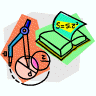## Mathematics, Department of## Faculty Publications, Department of Mathematics

7-7-2008

#### Citation

A universal theory of decoding and pseudocodewords; (with N. Axvig, D.T. Dreher, K. Morrison, E. Psota and L.C. Perez), SGER Technical Report 0801, University of Nebraska-Lincoln, March 2008.

#### Abstract

The discovery of turbo codes  and the subsequent rediscovery of low-density parity-check (LDPC) codes [9, 18] represent a major milestone in the field of coding theory. These two classes of codes can achieve realistic bit error rates, between 10−5 and 10−12, with signalto- noise ratios that are only slightly above the minimum possible for a given channel and code rate established by Shannon’s original capacity theorems. In this sense, these codes are said to be near-capacity-achieving codes and are sometimes considered to have solved (in the engineering sense, at least) the coding problem for the additive white Gaussian noise (AWGN) channel and its derivative channels. Perhaps the most important commonality between turbo and low-density parity-check codes is that they both utilize iterative message-passing decoding algorithms. For turbo codes, one uses the so-called turbo decoding algorithm, and for LDPC codes, both the sumproduct (SP) and the min-sum (MS) algorithms are used. The success of the various iterative message-passing algorithms is sometimes said to have ushered in a new era of “modern” coding theory in which the design emphasis has shifted from optimizing some code property, such as minimum distance, to optimizing the corresponding decoding structure of the code, such as the degree profile [24, 25], with respect to the behavior of a message-passing decoder. As successful as these codes and decoders have been in terms of application, there are several major questions that must be answered before a complete understanding of them can be achieved. The theoretical research in the area of capacity-achieving codes is focused on two main themes. The first theme is whether different types of capacity-achieving codes have common encoder and structural properties. In , it was claimed that turbo codes could be viewed as LDPC codes, but the relationship was not made explicit. More recently, P´erez, his student Jiang, and others [11, 16] developed a construction for the parity-check matrices of arbitrary turbo codes that clearly connects the components of the turbo encoder to the resulting structure of the parity-check matrix. From a more abstract perspective, turbo codes and low-density parity-check codes are examples of codes with long block lengths that exhibit the random structure inherent in Shannon’s original theorems. The second and more active research theme is the determination of the behavior of iterative message-passing decoding and the relationships between the various decoding algorithms. The dominant problem in this area is to understand the non-codeword decoder errors that occur in computer simulations of LDPC codes with iterative message-passing decoders. Motivated by empirical observations of the non-codeword outputs of LDPC decoders, the notion of stopping sets was first introduced by Forney, et al.  in 2001. Two years later, a formal definition of stopping sets was given by Changyan, et al. . They demonstrated that the bit and block error probabilities of iteratively decoded LDPC codes on the binary erasure channel (BEC) can be determined exactly from the stopping sets of the parity-check matrix. (Here, a stopping set S is a subset of the set of variable nodes such that all neighboring check nodes of S are connected to S at least twice.)

COinS